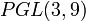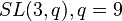# Projective special linear group:PSL(3,9)

View a complete list of particular groups (this is a very huge list!)[SHOW MORE]

## Definition

This group is a finite group defined in the following equivalent ways:

1. It is the projective special linear group of degree three over field:F9, denoted$PSL(3,9)$.
2. It is the special linear group of degree three over field:F9, denoted$SL(3,9)$.
3. It is the projective general linear group of degree three over field:F9, denoted$PGL(3,9)$.

### Equivalence of definitions

The equivalence of definitions follows from isomorphism between linear groups when degree power map is bijective.

## Arithmetic functions

### Basic arithmetic functions

Function Value Similar groups Explanation
order (number of elements, equivalently, cardinality or size of underlying set) 42456960 groups with same order As$SL(3,q), q = 9$:$q^3(q^3 - 1)(q^2 - 1) = 9^3(9^3 - 1)(9^2 - 1) = (729)(728)(80) = 42456960$
exponent of a group 21840 groups with same order and exponent of a group | groups with same exponent of a group
Frattini length 1 groups with same order and Frattini length | groups with same Frattini length As the group is a simple non-abelian group, its Frattini length must be one.

## Group properties

Property Satisfied? Explanation
abelian group No
nilpotent group No
solvable group No
simple group, simple non-abelian group Yes projective special linear group is simple
minimal simple group No contains projective special linear group:PSL(3,3). See classification of finite minimal simple groups.

## GAP implementation

### Descriptions

Description Functions used
PSL(3,9) PSL
SL(3,9) SL
PGL(3,9) PGL